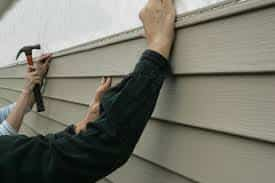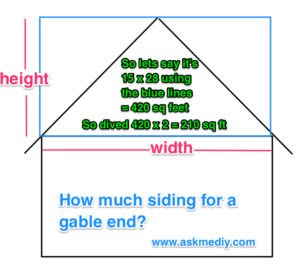## Figuring out the right amount of siding is actually very easy.

#### A few things to know

Vinyl siding comes in 12-foot lengths.All accessories also come in 12-foot lengths
Siding is sold by a “builder square”. Which is 100 square feet.

Two squares per box, for most types.

example: 10 feet by 10 feet.

So a whole box of siding is two squares or 200 square feet.

### How Much Siding For a Gable End? How to figure siding for gable end of home?

Total height X total width = ??? Divided by 2 = ?? simple right.Let’s say you have a Bi-level house and it’s 50 feet long. Without measuring, the 50 feet part is easy, but measuring the height is a little bit more involved. You need to know that you have eight-foot floor ceilings, right? Let’s do this: you have the floor joist for the first floor, the floor joist for the second floor and each one is about 12 inches x 2 plus your two levels (walls) @ 8 feet (96 inches)

2 floors (joist) = 2 feet

2 walls (levels) = 8 feet for a total height of 18 feet high.

So your house is 50 feet long by 18 feet high. Multiply those two numbers and you get 900 square feet.

Now you’re thinking, “but I have windows and a big sliding door…” And you’re right, but remember, the one thing you want to make sure of is that you have enough and you don’t want to start using little pieces towards the end of the job.

This is how I order. I get the total square footage and see what that comes to. Each box of siding comes with two squares in a box so you’re dealing with even numbers. If we have a total of 900 square feet, then you order ten squares. Which will be 1000 square feet and five boxes? Not 9 1/2. Remember, that 1/2 box is more likely to get damaged on delivery and hanging around until you use it, so it’s not worth not getting it. Once you’re done, you can always return full pieces using that long empty box that you now have.

On the other hand, let’s say you have a total of 825 square feet that you need. And you have some windows, and a door or two. Then I would order for 800 square feet (four boxes).

J channel. This is the trim that goes around the windows and doors. I like to order two pieces per window and three per door no matter how small or big.

In this example, I only showed you one wall. Chances are, you will be doing more than just one side of your house. Just use the same vinyl siding formula on the other walls.

Here is a website of mine for any tools you may need. TOOLS

1. You’ll need 120 sq feet. 1.2 square

2. I have a 10×12 shed , how much siding will I need ?

3. Tin? I don’t understand what you mean.

4. What size wood would I use over the tin 1×2 , 2×6 , or 2×4

5. 14x70x8 = 1,344 sq feet. So that would be 7 boxes 200 sq each box.

6. That would be 5 boxes

7. I’m sorry the walls are 8 feet high not 10 thank you

8. I have a 14x 70 mobile single wide so I would think that would be 980 sq ft how many boxes would I need with ten foot Wall’s

9. Maybe 15 box’s and maybe \$1,500. But yo have to know this is a loaded question.

10. how much boxes needed for 2800 square feet and the average price i would be looking at

11. How much for what? I have no idea I don’t see siding.

12. how many boxes of vinyl siding to cover 3100 sq ft and how much will it cost.

13. That’s for you to figure out. Read the whole post. 200 sq feet in a box.

14. I have a home that is 38’long,25’wide, 25’tall how many boxes of siding would I need to cover it?

15. You’ll need three boxes. Each one box holds 200 sq feet in most cases.

16. how many boxes of vinyl siding do I need for 483 square feet

17. You would not remove the tin. You would install wood strips over the tin.

18. I want to install vinyl siding on a single wide mobile home that is 12X60 and has tin on the outside right now. How much vinyl would I need and what do i put on the outside after i take the metal off the outside walls.

19. one half of a story. Same formula for figuring out your wall but think of it as one level of your house. So the total length of the wall by 9 ( an average wall height(8 feet) plus the average space between levels(1 foot) ) divided by 2 because the peak is one half of the square. But REMEMBER there are 2 sides so one half is likely not necessary.

20. how do YOU measure the ends of the house, figuring the triangle roof line?

21. Depending on how big the garage door is I would buy 4 box’s

22. I want to side my garage…one wall is 20’w x10’h so I figure that I need 200 sq ‘ x four walls or 800 sq ‘..I know I should subtract for one entry door and 1 car door.. since it comes in boxes … should I purchase 4 boxes?